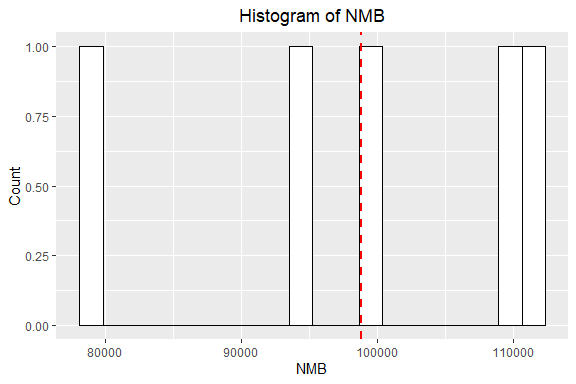Probabilistic sensitivity analysis

2021-03-02

In this example we will model the cost effectiveness of lamivudine/zidovudine combination therapy in HIV infection ([Chancellor, 1997] (https://pubmed.ncbi.nlm.nih.gov/10169387/)) and also described in Decision Modelling for Health Economic Evaluation, page 32. We have implemented the scenario in which the effect of combination therapy of lamivudine/zidovudine (as the probability of moving to worse health states and having to accrue more cost) is only seen in first 2 years. This means that the transition probability and costs are dependent on first two cycles (As the cycle length is years).

This model aims to compare costs and utilities of two treatment strategies, mono therapy and combined therapy.

Four states are described, from best to worst health-wise:

A: CD4 cells > 200 and < 500 cells/mm3; B: CD4 < 200 cells/mm3, non-AIDS; C: AIDS; D: Death.

library(packDAMipd)

Define health states for mono therapy - now the costs are just defined, but not defined any value.

A <- health_state("A", cost = "cost_health_A+ cost_drug ",utility = 1)
B <- health_state("B", cost = "cost_health_B + cost_drug",utility = 1)
C <- health_state("C", cost = "cost_health_C + cost_drug",utility = 1)
D <- health_state("D", cost = 0,utility = 0)

Define allowed transition probabilities and number them. The below matrix is numbered so that the maximum entry in the matrix gives the total number of allowed transitions. Column names and row names are just the names of the health states.

tmat <- rbind(c(1, 2,3,4), c(NA, 5,6,7),c(NA, NA, 8,9), c(NA, NA, NA,10))
colnames(tmat) <- rownames(tmat) <- c("A", "B" , "C", "D")

Define transition matrix now, but parameters are fine now

tm <- populate_transition_matrix(4, tmat, c("tpAtoA", "tpAtoB", "tpAtoC",
"tpAtoD",
"tpBtoB", "tpBtoC", "tpBtoD",
"tpCtoC", "tpCtoD", "tpDtoD" ),
colnames(tmat) )
>  "The transition matrix as explained"
>     transition number probability name from from state to to state
>  1:                 1      prob_A_to_A    1          A  1        A
>  2:                 2      prob_A_to_B    1          A  2        B
>  3:                 3      prob_A_to_C    1          A  3        C
>  4:                 4      prob_A_to_D    1          A  4        D
>  5:                 5      prob_B_to_B    2          B  2        B
>  6:                 6      prob_B_to_C    2          B  3        C
>  7:                 7      prob_B_to_D    2          B  4        D
>  8:                 8      prob_C_to_C    3          C  3        C
>  9:                 9      prob_C_to_D    3          C  4        D
> 10:                10      prob_D_to_D    4          D  4        D

Combine the health states and define the strategy. The current strategy ie. control or intervention - here it is mono therapy

health_states <- combine_state(A,B,C,D)
mono_strategy <- strategy(tm, health_states, "mono")

Before we run the model, we need to give values to parameters, thus we define the parameter list. We need to make sure that the parameters with numerical values are given first and derived parameters later in the list. The parameters are assigned sequentially as given in the parameter list. So if there is any calculations needed (or using functions), they are defined earlier.

mono_param_list = define_parameters(cost_zido = 2278,
cost_direct_med_A = 1701,
cost_comm_care_A = 1055,
cost_direct_med_B = 1774,
cost_comm_care_B = 1278,
cost_direct_med_C = 6948,
cost_comm_care_C = 2059,
tpAtoA = 1251/(1251 + 483),
tpAtoB = 350/(350 + 1384),
tpAtoC = 116/(116 + 1618),
tpAtoD = 17/(17 + 1717),
tpBtoB = 731/(731 + 527),
tpBtoC = 512/(512 + 746),
tpBtoD = 15/(15 + 1243),
tpCtoC = 1312/(1312 + 437),
tpCtoD = 437/(437 + 1312),
tpDtoD = 1,
cost_health_A = "cost_direct_med_A+ cost_comm_care_A",
cost_health_B = "cost_direct_med_B+ cost_comm_care_B",
cost_health_C = "cost_direct_med_C+ cost_comm_care_C",
cost_drug = "cost_zido")

We run the model with strategy mono_strategy for 20 cycles with initial state values 1,0,0,0 corresponding to A,B, C and D. Costs and quays are discounted following the values given in discount. Parameter values will have any parameter defined as health state values or transition probabilities, but not their values were assigned.

mono_markov <- markov_model(mono_strategy, 20,c(1,0,0,0),discount = c(0.06,0),mono_param_list, method = "half cycle correction",TRUE)

Now for combination therapy, the transition probabilities and cost are dependent on the years or the cycle number. Thus we need to define functions that given the correct output to calculate the probabilities and costs as a function of cycle

#Define function to set the cost to be different for first two cycles
define_comb_cost = function(cycle,cost_lami) {
if (cycle == 2 || cycle == 3 )
return(cost_lami)
else
return(0)
}
#Define function to set the risk ratio to be different for first two cycles

define_rr = function(cycle,rr) {
if (cycle == 2 || cycle == 3)
return(rr)
else
return(1)
}

Define health states - now the costs are just defined, but not defined any value.

A <-  health_state("A", cost = "cost_health_A + cost_drug", utility = 1)
B <- health_state("B", cost = "cost_health_B + cost_drug", utility = 1)
C <- health_state("C", cost = "cost_health_C + cost_drug", utility = 1)
D <- health_state("D", cost = 0, utility = 0)

Define allowed transition probabilities and number them. The below matrix is numbered so that the maximum entry in the matrix gives the total number of allowed transitions. Column names and row names are just the names of the health states.

tmat <- rbind(c(1, 2,3,4), c(NA, 5,6,7),c(NA, NA, 8,9), c(NA, NA, NA,10))
colnames(tmat) <- rownames(tmat) <- c("A", "B" , "C", "D")

Define transition matrix now, but parameters are fine now

tm <- populate_transition_matrix(4, tmat, c("tpAtoA_rr", "tpAtoB_rr",
"tpAtoC_rr", "tpAtoD_rr",
"tpBtoB_rr", "tpBtoC_rr", "tpBtoD_rr",
"tpCtoC_rr", "tpCtoD_rr", "tpDtoD_rr" ), colnames(tmat) )
>  "The transition matrix as explained"
>     transition number probability name from from state to to state
>  1:                 1      prob_A_to_A    1          A  1        A
>  2:                 2      prob_A_to_B    1          A  2        B
>  3:                 3      prob_A_to_C    1          A  3        C
>  4:                 4      prob_A_to_D    1          A  4        D
>  5:                 5      prob_B_to_B    2          B  2        B
>  6:                 6      prob_B_to_C    2          B  3        C
>  7:                 7      prob_B_to_D    2          B  4        D
>  8:                 8      prob_C_to_C    3          C  3        C
>  9:                 9      prob_C_to_D    3          C  4        D
> 10:                10      prob_D_to_D    4          D  4        D

Before we run the model, we need to give values to parameters, thus we define the parameter list.

comb_param_list <- define_parameters(cost_zido = 2278,
cost_direct_med_A = 1701,
cost_comm_care_A = 1055,
cost_direct_med_B = 1774,
cost_comm_care_B = 1278,
cost_direct_med_C = 6948,
cost_comm_care_C = 2059,
tpAtoA = 1251/(1251 + 483),
tpAtoB = 350/(350 + 1384),
tpAtoC = 116/(116 + 1618),
tpAtoD = 17/(17 + 1717),
tpBtoB = 731/(731 + 527),
tpBtoC = 512/(512 + 746),
tpBtoD = 15/(15 + 1243),
tpCtoC = 1312/(1312 + 437),
tpCtoD = 437/(437 + 1312),
tpDtoD = 1,
rr = 0.509,
cost_lami = 2086.50,
rr_cycle = "define_rr(markov_cycle,rr)",
tpAtoA_rr = "1-tpAtoB*rr_cycle-tpAtoC*rr_cycle-
tpAtoD*rr_cycle",
tpAtoB_rr = "tpAtoB*rr_cycle",
tpAtoC_rr = "tpAtoC*rr_cycle",
tpAtoD_rr = "tpAtoD*rr_cycle",
tpBtoB_rr = "1-tpBtoC*rr_cycle-tpBtoD*rr_cycle",
tpBtoC_rr = "tpBtoC*rr_cycle",
tpBtoD_rr = "tpBtoD*rr_cycle",
tpCtoC_rr = "1-tpCtoD*rr_cycle",
tpCtoD_rr = "tpCtoD*rr_cycle",
tpDtoD_rr = 1,
cost_health_A = "cost_direct_med_A + cost_comm_care_A",
cost_health_B = "cost_direct_med_B + cost_comm_care_B",
cost_health_C = "cost_direct_med_C + cost_comm_care_C",
cost_lami_cycle = "define_comb_cost(markov_cycle,cost_lami)",
cost_drug = "cost_zido + cost_lami_cycle")
# Combine the health states
health_states <- combine_state(A,B,C,D)

#The current strategy ie. control or intervention - here it is combination
#therapy
comb_strategy <- strategy(tm, health_states, "comb")

We run the model with strategy mono_strategy for 20 cycles with initial state values 1,0,0,0 corresponding to A,B, C and D. Costs and quays are discounted following the values given in discount. Parameter values will have any parameter defined as health state values or transition probabilities, but not their values were assigned

comb_markov <- markov_model(comb_strategy, 20, c(1, 0,0,0), discount = c(0.06,0.0),comb_param_list, method = "half cycle correction", TRUE)

Now we will define the parameters for the sensitivity analysis. The parameters are already defined as mono_param_list and comb_param_list. The parameter with sampling distributions are defined using definer_parameters() and create parameter table using define_parameters_psa(). Then probabilistic sensitivity analysis is done for the Markov model of the choice here the mono_Markov using the function do_psa Using the result obtained, a full report or plot (for specific variable) can be generated using summary_plot_psa(). To get a report or plot of sensitivity analysis on ICER on NMB, two Markov models need to be passed on.

sample_list <- define_parameters(rr = "lognormal(mean = 0.509, sd = 0.173)",
cost_comm_care_C = "gamma(mean = 2756, sd = sqrt(2756))")
param_table_mono <- define_parameters_psa(mono_param_list, sample_list)
param_table_combo <- define_parameters_psa(comb_param_list, sample_list)

result_psa_mono = do_psa(mono_markov, param_table_mono, 5)
result_psa_comb = do_psa(comb_markov, param_table_combo, 5)

list_result_psa_mono <- list_paramwise_psa_result(result_psa_mono, NULL, NULL,
NULL)
list_result_psa_comb <- list_paramwise_psa_result(result_psa_comb, NULL, NULL,
NULL)
list_all <- list_paramwise_psa_result(result_psa_mono,result_psa_comb, 20000,
"mono")

summary_plot_psa(result_psa_mono, NULL, NULL, NULL)
> $mean_cost >  48769.59 > >$mean_utility
>  8.475325
>
> $sd_cost >  117.9465 > >$sd_utility
>  0
>
> $cost_utility_plot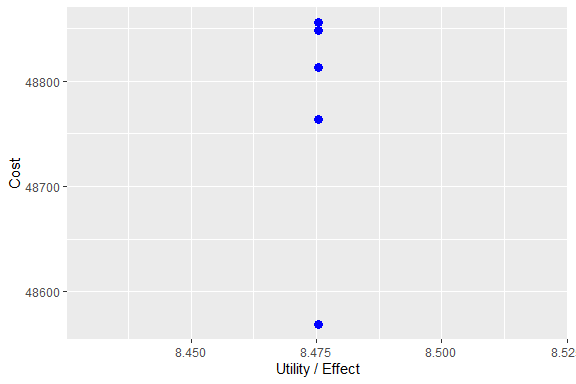> >$hist_cost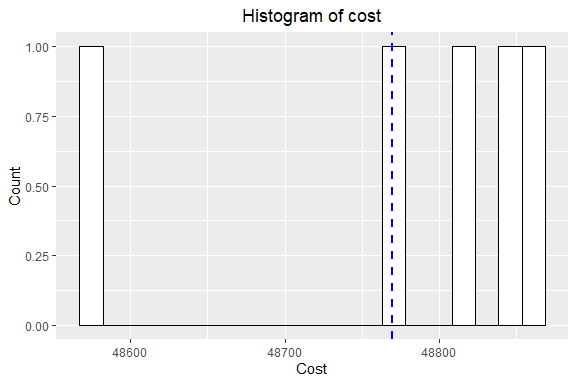>
> $hist_utility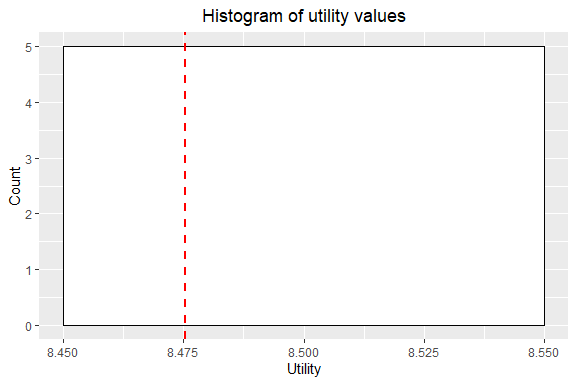summary_plot_psa(result_psa_comb, NULL, NULL, NULL) >$mean_cost
>  46297.36
>
> $mean_utility >  7.255503 > >$sd_cost
>  3001.002
>
> $sd_utility >  0.8041252 > >$cost_utility_plot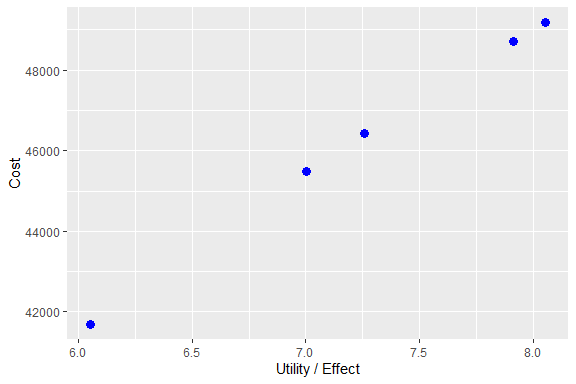>
> $hist_cost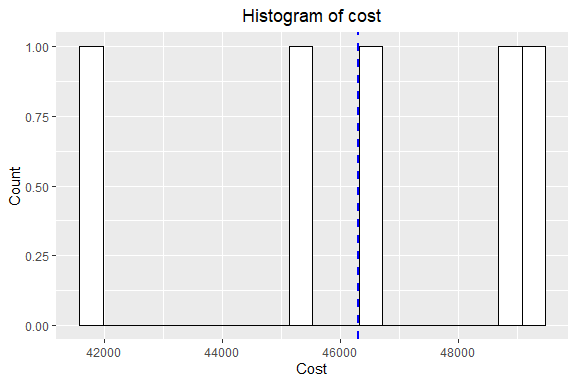> >$hist_utility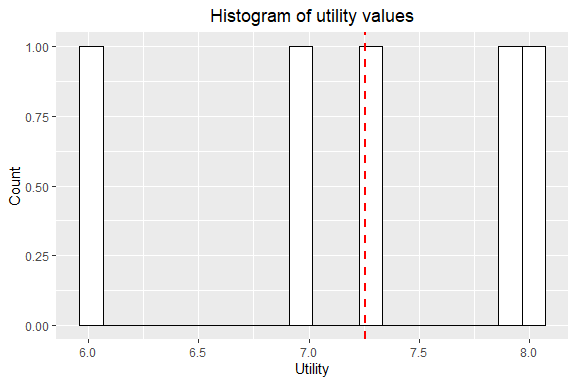summary_plot_psa(result_psa_mono,result_psa_comb, 20000, "mono")
> $mean_icer >  1168.571 > >$mean_nmb
>  98812.7
>
> $sd_icer >  1810.926 > >$sd_nmb
>  13084.35
>
> $icer_nmb_plot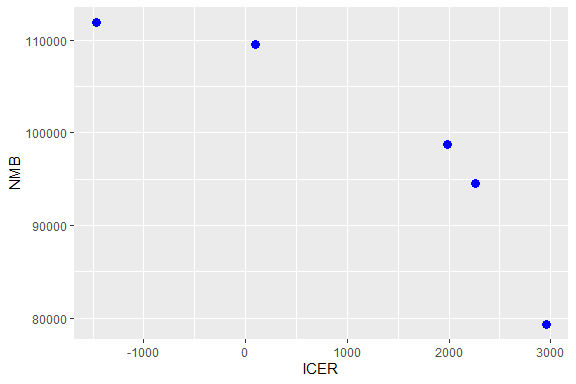> >$hist_icer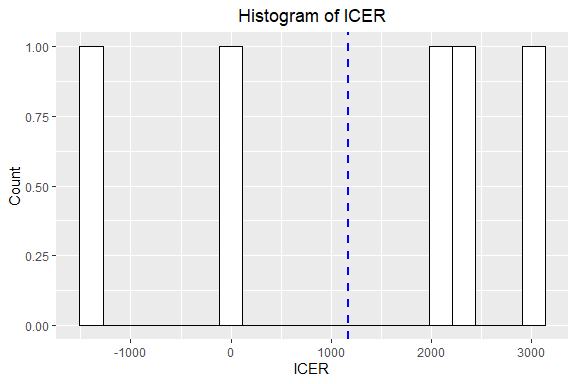>
> \$hist_nmb